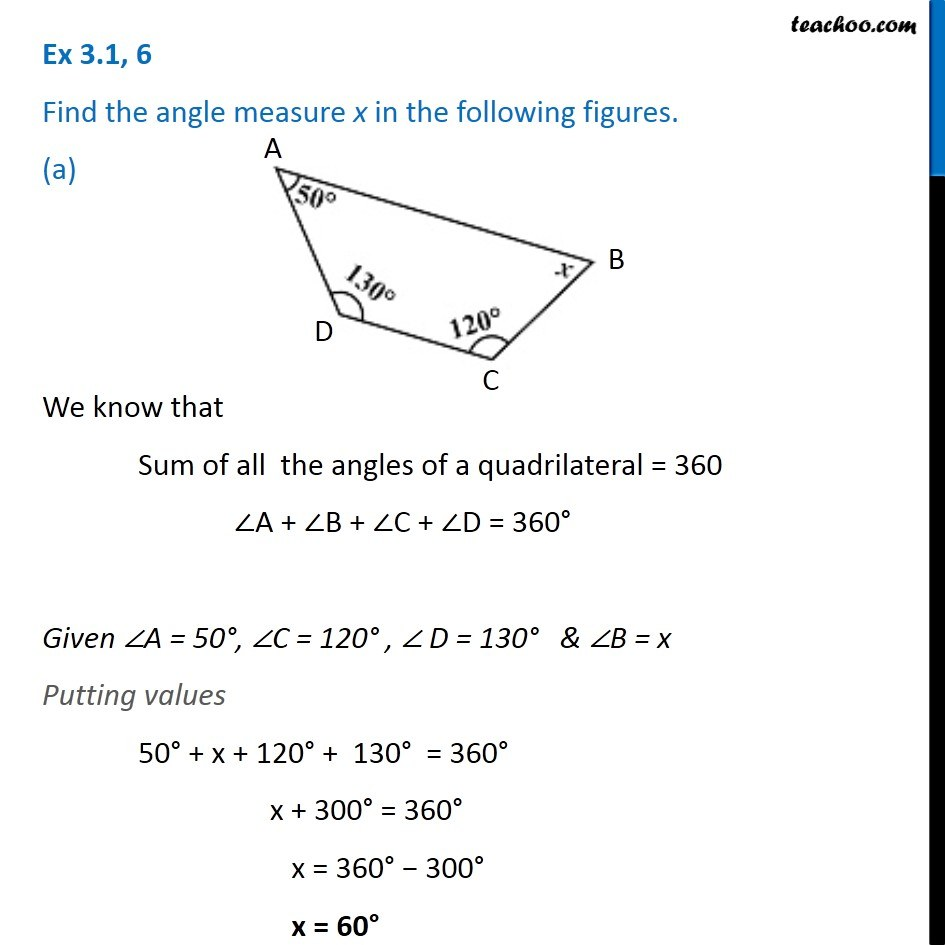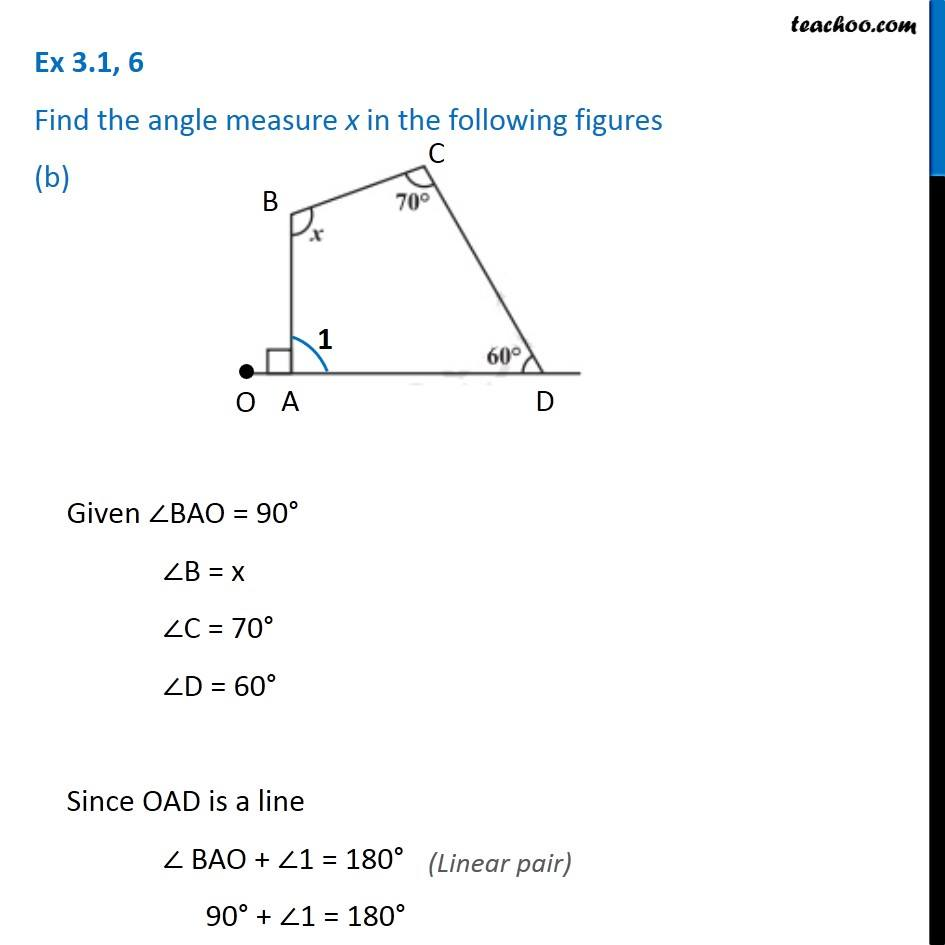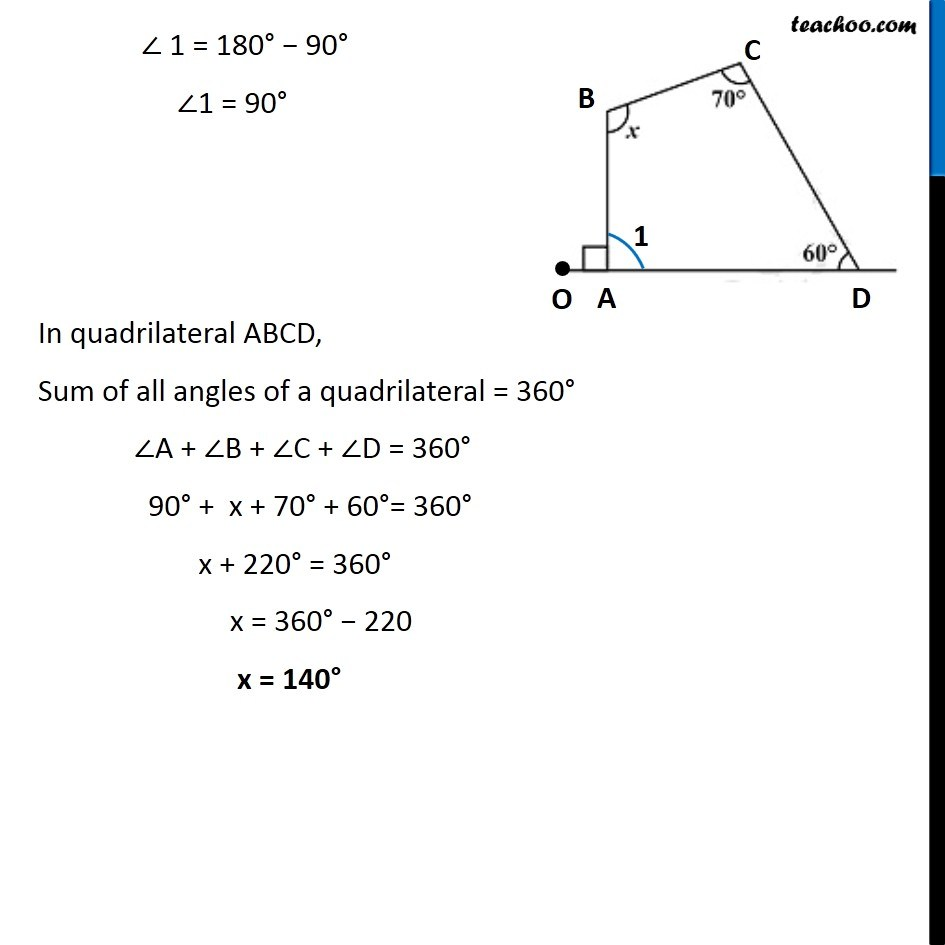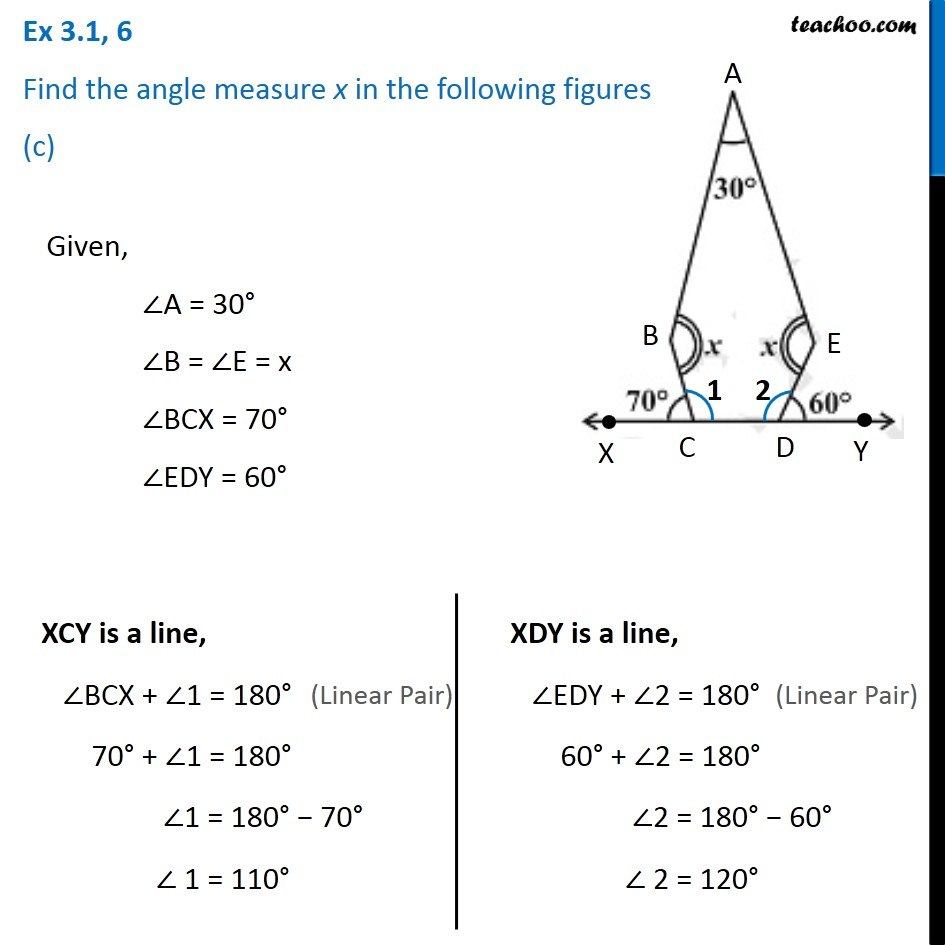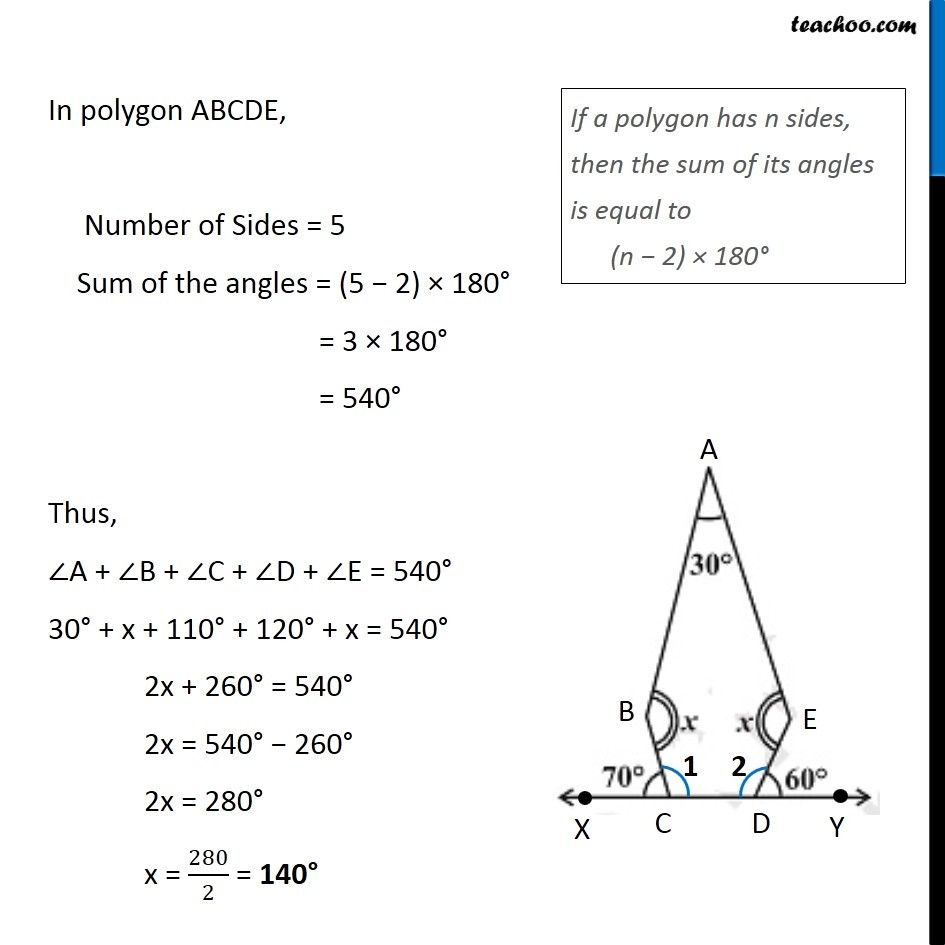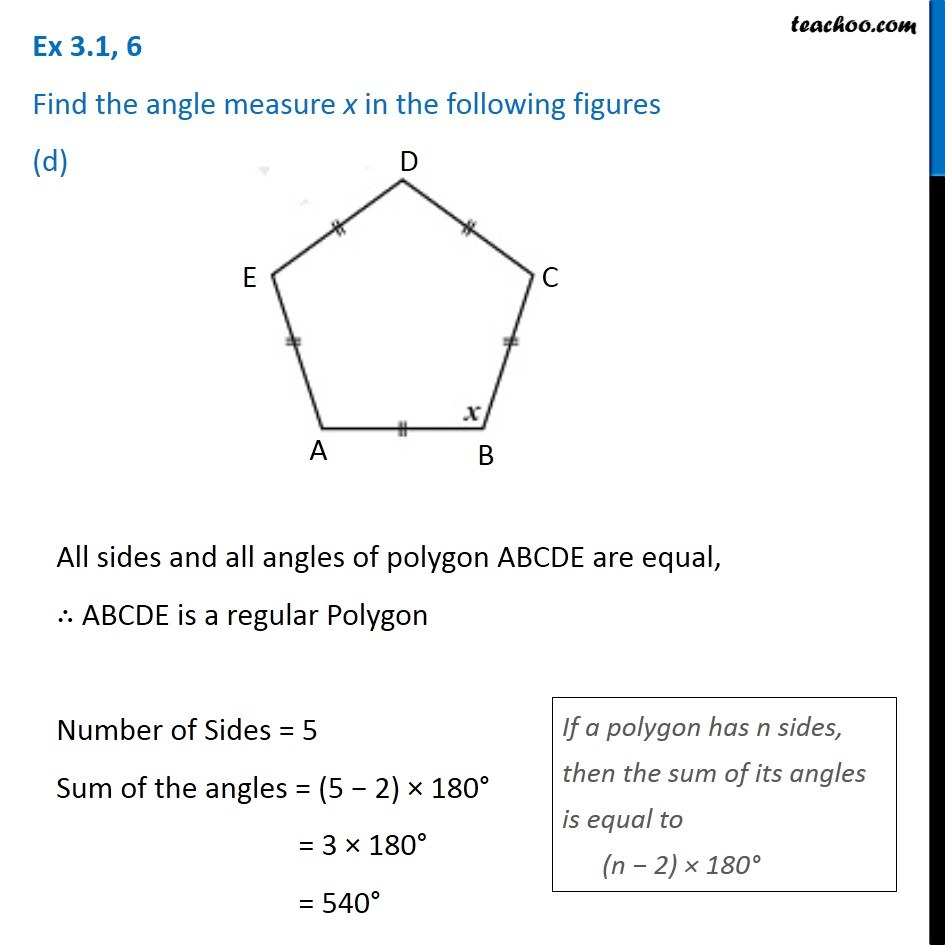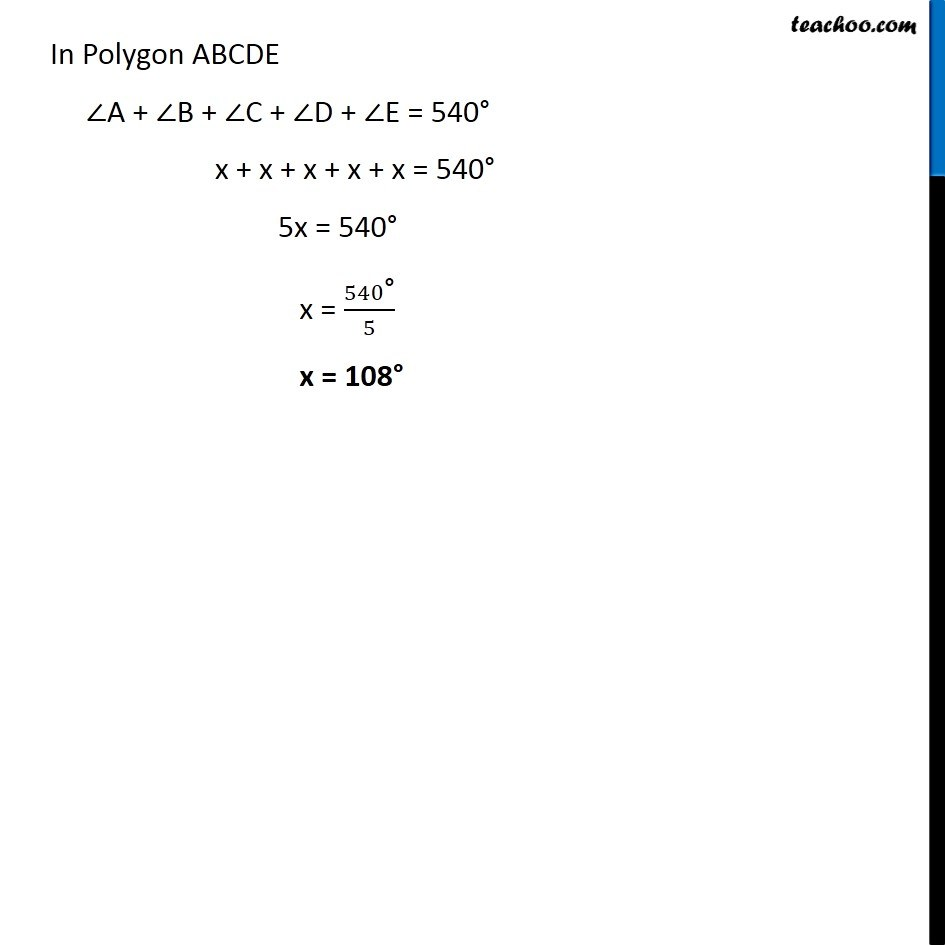Subscribe to our Youtube Channel - https://you.tube/teachoo

1. Chapter 3 Class 8 Understanding Quadrilaterals
2. Concept wise
3. Polygons

Transcript

Ex 3.1, 6 Find the angle measure x in the following figures. (a) We know that Sum of all the angles of a quadrilateral = 360 ∠A + ∠B + ∠C + ∠D = 360° Given ∠A = 50°, ∠C = 120° , ∠ D = 130° & ∠B = x Putting values 50° + x + 120° + 130° = 360° x + 300° = 360° x = 360° − 300° x = 60° Ex 3.1, 6 Find the angle measure x in the following figures (b) Given ∠BAO = 90° ∠B = x ∠C = 70° ∠D = 60° Since OAD is a line ∠ BAO + ∠1 = 180° 90° + ∠1 = 180° ∠ 1 = 180° − 90° ∠1 = 90° In quadrilateral ABCD, Sum of all angles of a quadrilateral = 360° ∠A + ∠B + ∠C + ∠D = 360° 90° + x + 70° + 60°= 360° x + 220° = 360° x = 360° − 220 x = 140° Ex 3.1, 6 Find the angle measure x in the following figures (c)Given, ∠A = 30° ∠B = ∠E = x ∠BCX = 70° ∠EDY = 60° XCY is a line, ∠BCX + ∠1 = 180° 70° + ∠1 = 180° ∠1 = 180° − 70° ∠ 1 = 110° XDY is a line, ∠EDY + ∠2 = 180° 60° + ∠2 = 180° ∠2 = 180° − 60° ∠ 2 = 120° In polygon ABCDE, Number of Sides = 5 Sum of the angles = (5 − 2) × 180° = 3 × 180° = 540° Thus, ∠A + ∠B + ∠C + ∠D + ∠E = 540° 30° + x + 110° + 120° + x = 540° 2x + 260° = 540° 2x = 540° − 260° 2x = 280° x = 280/2 = 140° If a polygon has n sides, then the sum of its angles is equal to (n − 2) × 180° Ex 3.1, 6 Find the angle measure x in the following figures (d) All sides and all angles of polygon ABCDE are equal, ∴ ABCDE is a regular Polygon Number of Sides = 5 Sum of the angles = (5 − 2) × 180° = 3 × 180° = 540° If a polygon has n sides, then the sum of its angles is equal to (n − 2) × 180° In Polygon ABCDE ∠A + ∠B + ∠C + ∠D + ∠E = 540° x + x + x + x + x = 540° 5x = 540° x = 540"°" /5 x = 108°

Polygons